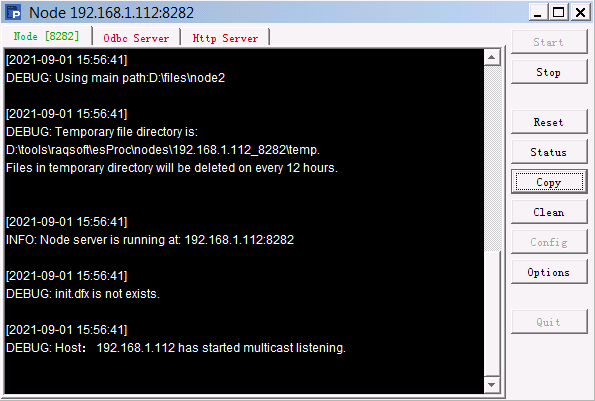# 坐标系与转换

## 物理轴与逻辑轴

Ø  按比例设定

Ø  按像素设定

 A 1 =canvas() 2 =A1.plot("BackGround") 3 =A1.plot("NumericAxis","autoCalcValueRange":false) 4 =A1.draw@p(450,350)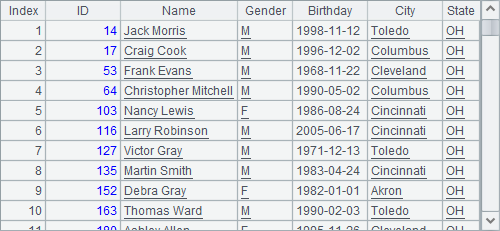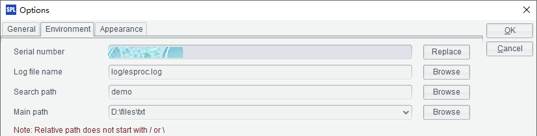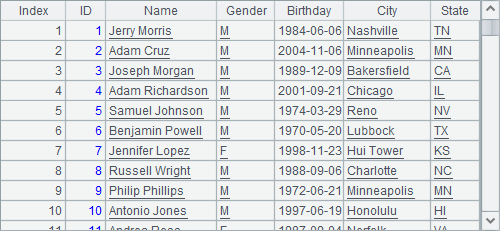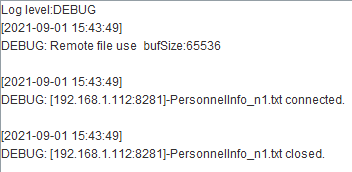X endY position被更改为了大于1的值，处理这两个属性时，物理轴的坐标原点仍然为左上角，但是将按像素设定，绘图结果如下：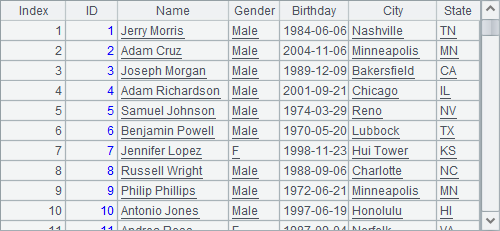X end400，即横轴终止点距离画布左侧400个像素。按像素设定物理轴坐标，可以使绘图更为精确，但是不便于绘制画布尺寸不定的图形。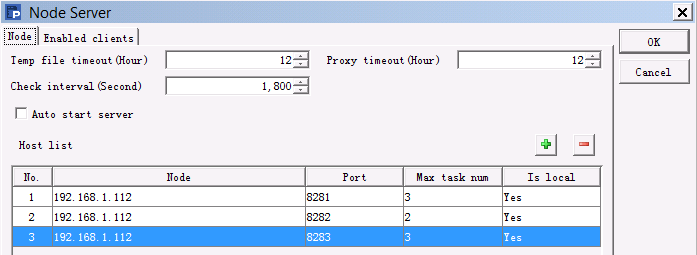X endY position更改为负值，绘图结果如下：A 1 =canvas() 2 =A1.plot("BackGround") 3 =A1.plot("NumericAxis","name":"x","autoCalcValueRange":false) 4 =A1.plot("NumericAxis","name":"y","location":2,"autoCalcValueRange": false) 5 6 =A1.draw@p(350,200)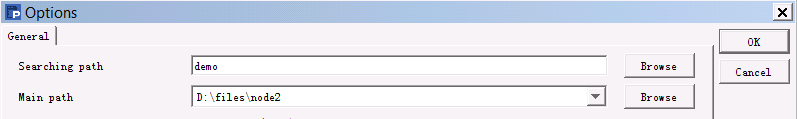A5中添加绘制点形图：

 A 1 =canvas() 2 =A1.plot("BackGround") 3 =A1.plot("NumericAxis","name":"x","autoCalcValueRange":false) 4 =A1.plot("NumericAxis","name":"y","location":2,"autoCalcValueRange": false) 5 =A1.plot("Dot","axis1":"x","data1":"2","axis2":"y","data2":"6") 6 =A1.draw@p(350,200)

A5中点形图的绘图参数如下：